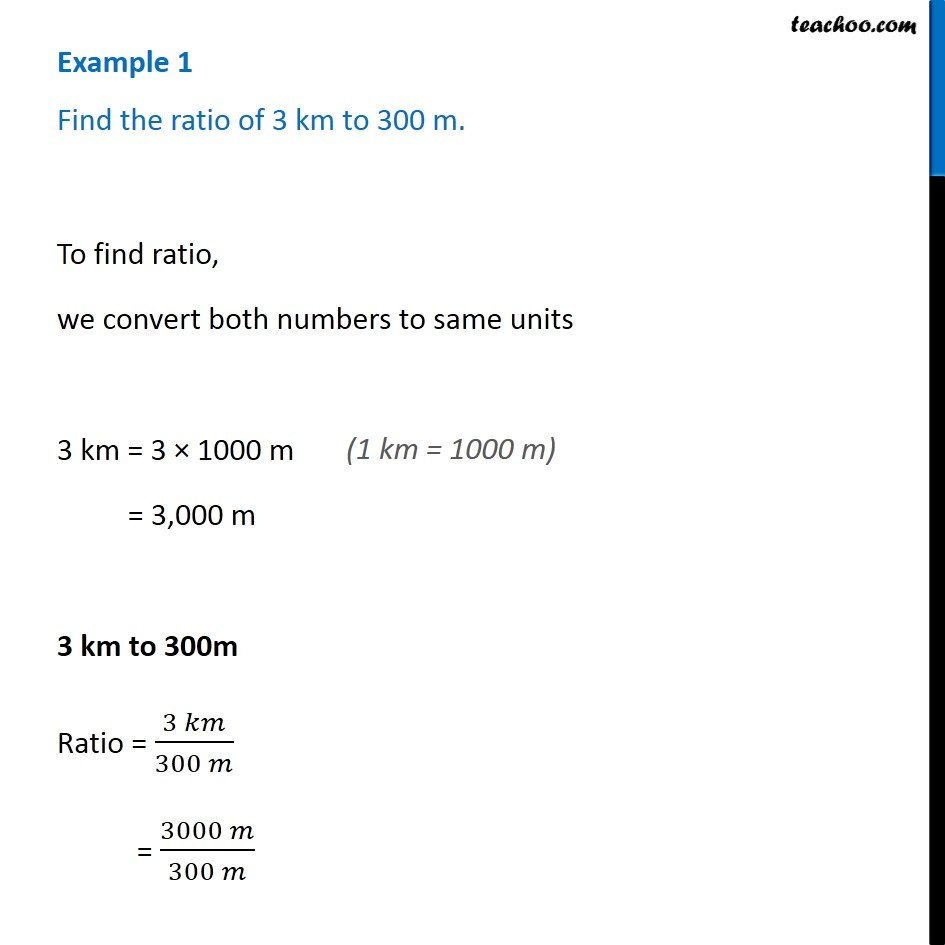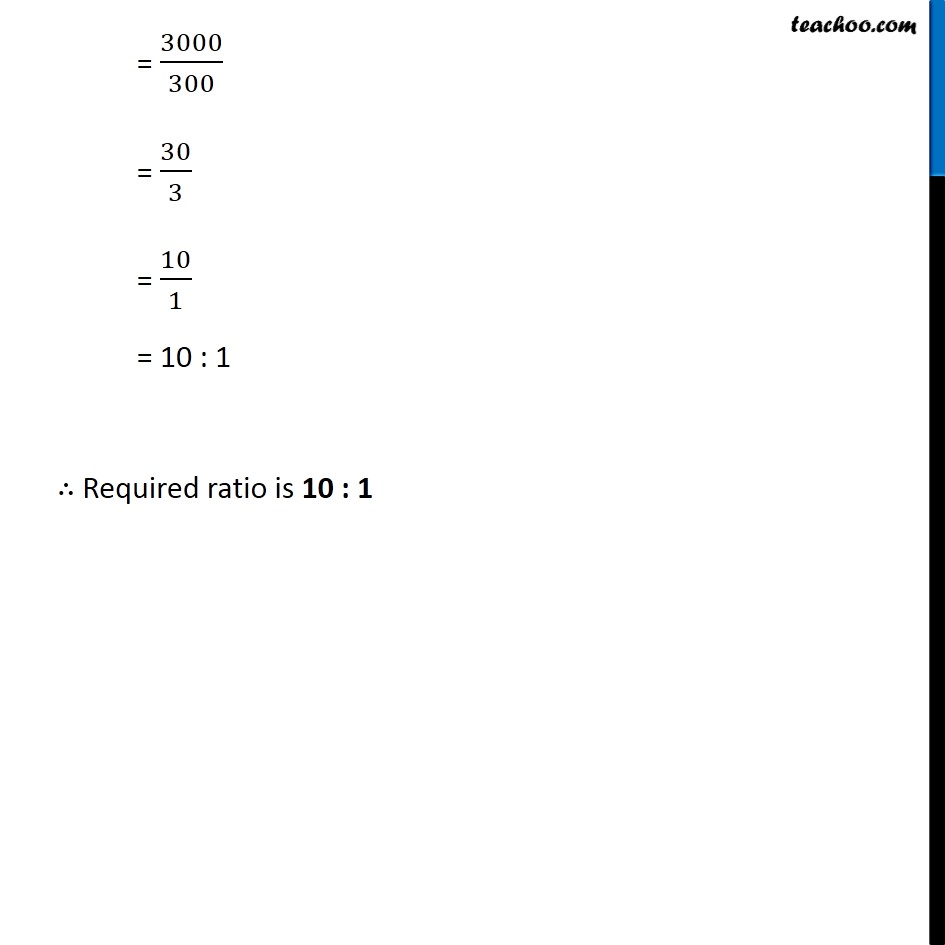Ratios

Chapter 8 Class 7 Comparing Quantities
Concept wiseIntroducing your new favourite teacher - Teachoo Black, at only ₹83 per month

### Transcript

Example 1 Find the ratio of 3 km to 300 m. To find ratio, we convert both numbers to same units 3 km = 3 × 1000 m = 3,000 m 3 km to 300m Ratio = (3 𝑘𝑚)/(300 𝑚) = (3000 𝑚)/(300 𝑚) = 3000/300 = 30/3 = 10/1 = 10 : 1 ∴ Required ratio is 10 : 1